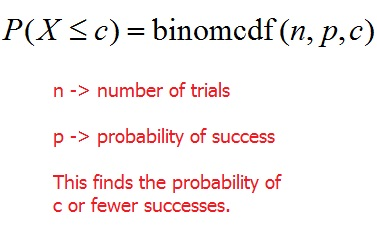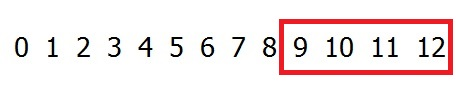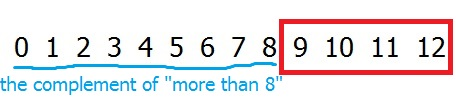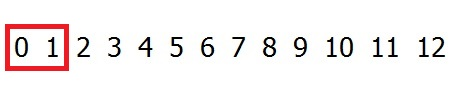﻿ Binomial probabilities - examples (calculator) - MathBootCamps

# Binomial probabilities – examples (calculator)

Once you have determined that an experiment is a binomial experiment, then you can apply either the formula or technology (like a TI calculator) to find any related probabilities. In this lesson, we will work through an example using the TI 83/84 calculator. If you aren’t sure how to use this to find binomial probabilities, please check here: Details on how to use a calculator to find binomial probabilities.

## Example

A student is taking a multiple choice quiz but forgot to study and so he will randomly guess the answer to each question. There are a total of 12 questions, each with 4 answer choices. Only one answer is correct for each question.

### Verifying the experiment is binomial

We know that this experiment is binomial since we have $$n = 12$$ trials of the mini-experiment “guess the answer on a question”. There are two outcomes: “guess correctly”, “guess incorrectly”.

If we treat a success as guessing a question correctly, then since there are 4 answer choices and only 1 is correct, the probability of success is:

$$p = \dfrac{1}{4} = 0.25$$

Finally, since the guessing is random, it is reasonable to assume that each guess is independent of the other guesses.

### Calculating probabilities

We will let $$X$$ represent the number of questions guessed correctly. Let’s now use this binomial experiment to answer a few questions.

(a) Find the probability that he answers 6 of the questions correctly.

This is asking for the probability of 6 successes, or $$P(X = 6)$$. For finding an exact number of successes like this, we should use binompdf from the calculator.

For this problem, $$n = 12$$ and $$p = 0.25$$. Therefore:

\begin{align} P(X=6) &= \text{binompdf(12,0.25,6)} \\ &\approx \boxed{0.0401}\end{align}

(b) Find the probability that he correctly answers 3 or fewer of the questions.

The probability of 3 or fewer successes is represented by $$P(X < 3)$$. Anytime you are counting down from some possible value of $$X$$, you will use binomcdf.\begin{align}P(X < 3) &= \text{binomcdf(12, 0.25, 3)} \\ &\approx \boxed{0.6488}\end{align}

It isn’t looking good. This is a pretty high chance that the student only answers 3 or fewer correctly!

(c) Find the probability that he correctly answers more than 8 questions.

This probability is represented by $$P(X > 8)$$. To understand how to find this probability using binomcdf, it is helpful to look at the following diagram.This shows all possible values of $$X$$ with the values which would represent “more than 8 successes” highlighted in red. To calculate this, we could do the binompdf of 9, the binompdf of 10, the binompdf of 11, and the binompdf of 12 and add them all together. But, this would take quite a while. Instead, we could use the complementary event.Recall that $$P(A)$$ is $$1 – P(A \text{ complement})$$. So, we can write:

\begin{align} P(X > 8) &= 1 – P( X < 8) \\ &= 1 - \text{binomcdf(12, 0.25, 8)}\\ &\approx \boxed{3.9 \times 10^{-4}}\end{align}

This is a very small probability. Looks like the random guessing probably won’t pay off too much.

(d) Find the probability that he correctly answers 5 or more questions.

This probability is represented by $$P(X \geq 5)$$. Using our diagram:Again, since this is asking for a probability of > or $$\geq$$;, and the CDF only counts down, we will use the complement. Notice that the complementary event starts with 4 and counts down. So, we will use 4 in the CDF.

\begin{align}P(X \geq 5) &= 1 – P(X < 5)\\ &= 1 - \text{binomcdf(12, 0.25, 4)}\\ &\approx \boxed{0.1576}\end{align}

(e) Find the probability that he correctly answers fewer than 2 questions.

This is asking for $$P(X < 2)$$.Since this is counting down, we can use binomcdf. But, the event “fewer than 2” does not include 2. So, we will put 1 into the cdf function.

\begin{align} P(X < 2) &= \text{binomcdf(12, 0.25, 1)}\\ &\approx \boxed{0.1584}\end{align}

(e) Find the probability that he correctly answers between 5 and 10 questions (inclusive) correctly.

Since this is inclusive, we are including the values of 5 and 10. That is, we are finding $$P(5 \leq X \leq 10)$$.Remember, you can always find the PDF of each value and add them up to get the probability. Here however, we can creatively use the CDF. Recall that the CDF takes whatever value you put in and adds the PDFs for each value starting with that number all the way down to zero. If we find the CDF of 10, it will add the PDFs of 10, 9, 8, 7, 6, 5, 4, 3, 2, 1, and 0.This will include all the values below 5, which we don’t want. So, we will subtract them out!This will leave exactly the values we want:

\begin{align}P(5 \leq X \leq 10) &= \text{binomcdf(12,0.25,10)} – \text{binomcdf(12,0.25,4)}\\ &\approx \boxed{0.1576}\end{align}

## Interesting observation

Did you notice that two of our answers were really similar? We found that:

\begin{align}P(X \geq 5) &= 1 – P(X < 5)\\ &= 1 - \text{binomcdf(12, 0.25, 4)}\\ &\approx \boxed{0.1576}\end{align}

and

\begin{align}P(5 \leq X \leq 10) &= \text{binomcdf(12,0.25,10)} – \text{binomcdf(12,0.25,4)}\\ &\approx \boxed{0.1576}\end{align}

What is going on here?

Well, these probabilities aren’t exactly the same. The first is actually 0.1576436761 while the second is 0.1576414707. These are certainly very close though! The tiny difference is because $$P(X \geq 5)$$ includes $$P(X = 11)$$ and $$P(X = 12)$$, while $$P(5 \leq X \leq 10)$$ does not.

Further, $$P(X = 11)$$ represents the probability that he correctly answers 11 of the questions correctly and latex $$P(X = 12)$$ represents the probability that he answers all 12 of the questions correctly. Both events are very unlikely since he is guessing! In fact:

\begin{align}P(X = 11) &= \text{binompdf(12,0.25,11)} \\ &\approx \boxed{2.14 \times 10^{-6}}\end{align}

and

\begin{align} P(X = 12) &= \text{binompdf(12,0.25,12)} \\ &\approx \boxed{5.96 \times 10^{-8}}\end{align}

Since these are so tiny, including them in the first probability only increases the probability a little bit. Rounding to 4 decimal places, we didn’t even catch the difference.

## Summary

The only reason we were able to calculate these probabilities is because we recognized that this was a binomial experiment. This fact allowed us to use binompdf for exact probabilities and binomcdf for probabilities that included multiple values. Just remember – binomcdf is cumulative. It adds up PDFs for the value you put in, all the way down to zero. Using this, you can find pretty much any binomial probability as long as you use something like the diagrams we drew above to keep track of the needed values.## Subscribe to our Newsletter!

We are always posting new free lessons and adding more study guides, calculator guides, and problem packs.

Sign up to get occasional emails (once every couple or three weeks) letting you know what's new!